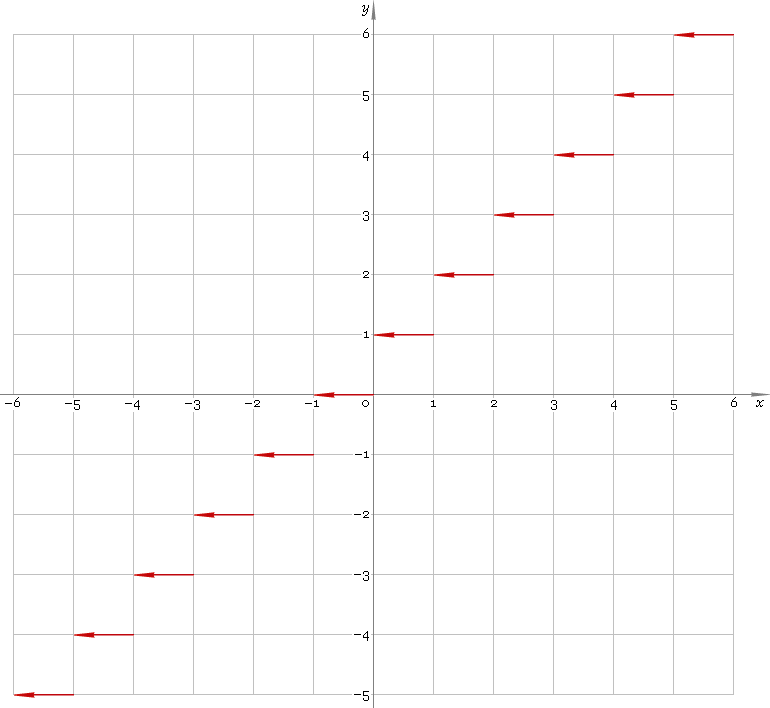The Art of Mathematics

# ceil — ceiling function

## 1. Definition

Ceiling is the nearest integer to the righ — smallest integer greater than or equal to the argument.

## 2. Graph

Ceiling function defined everywhere on real axis — so, its domain is (−∞, +∞). Its stair-like graph is depicted below — fig. 1.Fig. 1. Graph of the ceiling function y = ceilx.

Function codomain is the set of integer numbers.

## 3. How to use

To calculate ceiling of the number:

``ceil(−1.8);``

To get ceiling of the complex number:

``ceil(−1.8+i*1.2);``

To get ceiling of the current result:

``ceil(rslt);``

To get ceiling of the number z in calculator memory:

``ceil(mem[z]);``

## 5. Support

Ceiling function of the complex argument is supported in professional version of the Librow calculator.